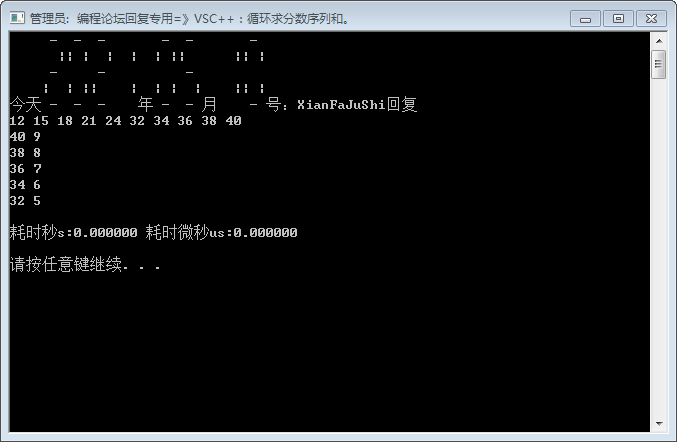2021-05-10 16:01

# C语言向一维数组a中输入10个数据：12，15，18，21，24，32，34，36，38，4

C语言向一维数组a中输入10个数据：12，15，18，21，24，32，34，36，38，40，求出这十个数据的平均值，并输入高于平均值的所有元素值及下标
• 点赞
• 写回答
• 关注问题
• 收藏
• 邀请回答

#### 3条回答默认 最新

•智者知已应修善业 2021-05-10 16:18
``````int a{},j=0,n=0;
while(n<10)std::cin>>a[n],j+=a[n],++n;
j/=10;
while(--n)if(a[n]>j)std::cout<<a[n]<<ends<<n<<endl;``````
``````	double a{}, j = 0;int n = 0;
while (n<10)std::cin >> a[n], j += (a[n]*=1.0), ++n;
j /= 10;
while (--n)if (a[n]>j)std::cout << a[n] << ends << n << endl;``````点赞 1 评论
•benbenli 2021-05-10 22:58
``````
#include <stdio.h>

int main()
{
int a = {12, 15, 18, 21, 24, 32, 34, 36, 38, 40};

int sum = 0;
for (int i = 0; i < 10; ++i)
sum += a[i];

float average = sum / 10.0;
printf("Average: %f\n", average);

printf("Index and number bigger than average:\n");
for (int i = 0; i < 10; ++i)
if (a[i] > average)
printf("index %d, number %d\n", i, a[i]);
}

// Output
Average: 27.000000
Index and number bigger than average:
index 5, number 32
index 6, number 34
index 7, number 36
index 8, number 38
index 9, number 40  ``````

点赞 1 评论
•Kscstg 2021-05-11 09:45

# include <stdio.h>

int main()
{
int a = {12, 15, 18, 21, 24, 32, 34, 36, 38, 40 };
int sum = 0;
int i = 0；
for (i = 0; i < 10; i++)
sum = sum + a[i];

double average = sum * 1.0 / 10;
printf("平均数为 %lf\n", average);

printf("大于平均值的数有:\n");
for (i = 0; i < 10; i++)
{
if (a[i] > average)
{
printf("下标 %d, 值为 %d ", i, a[i]);
}

}
return 0;
}

点赞 1 评论